Miscellaneous

Chapter 5 Class 11 Complex Numbers
Serial order wise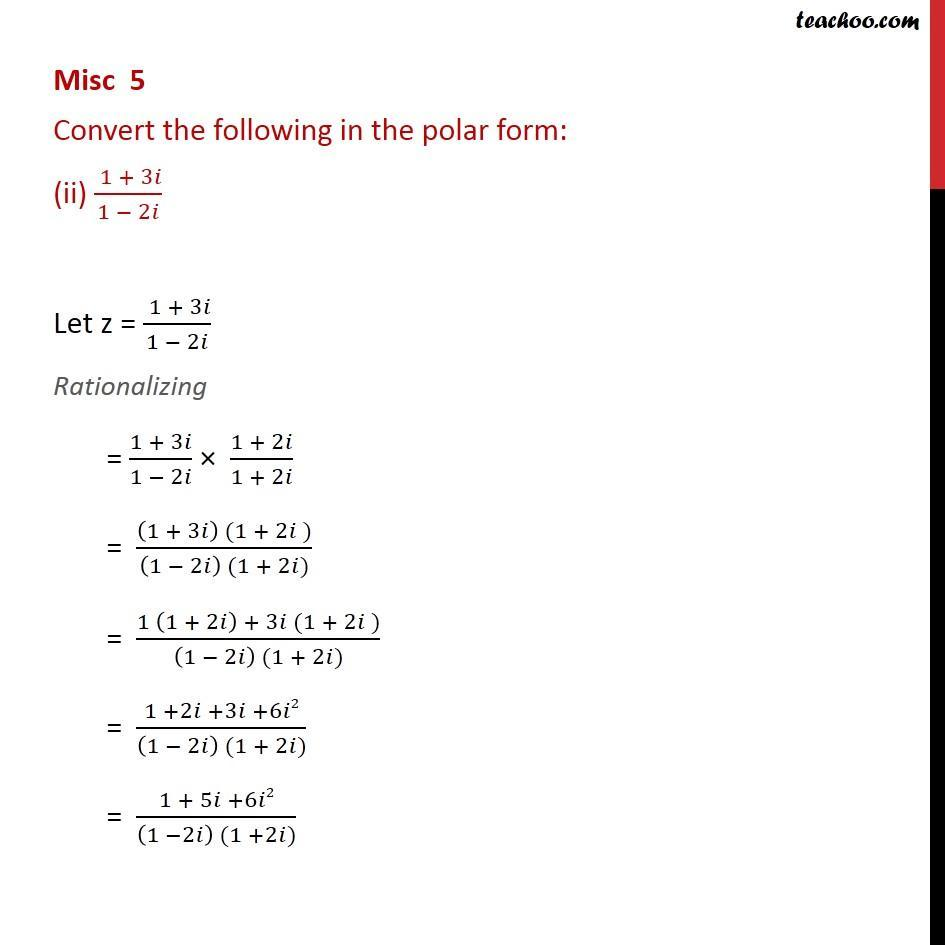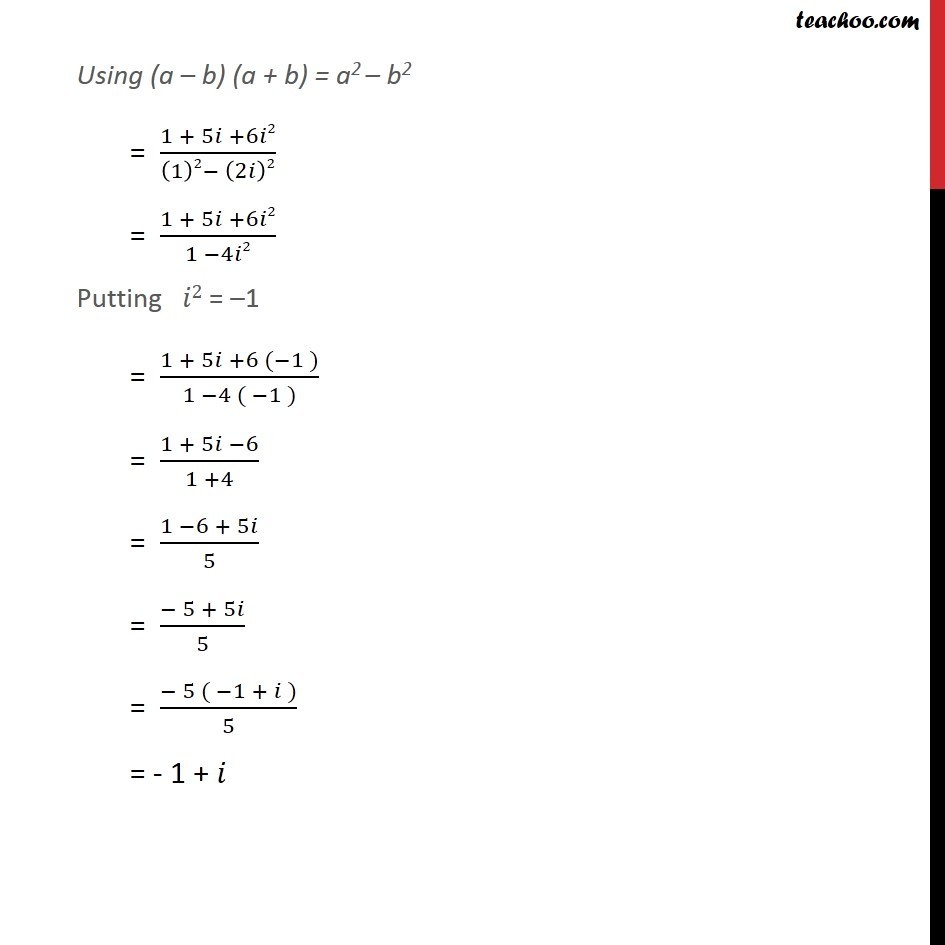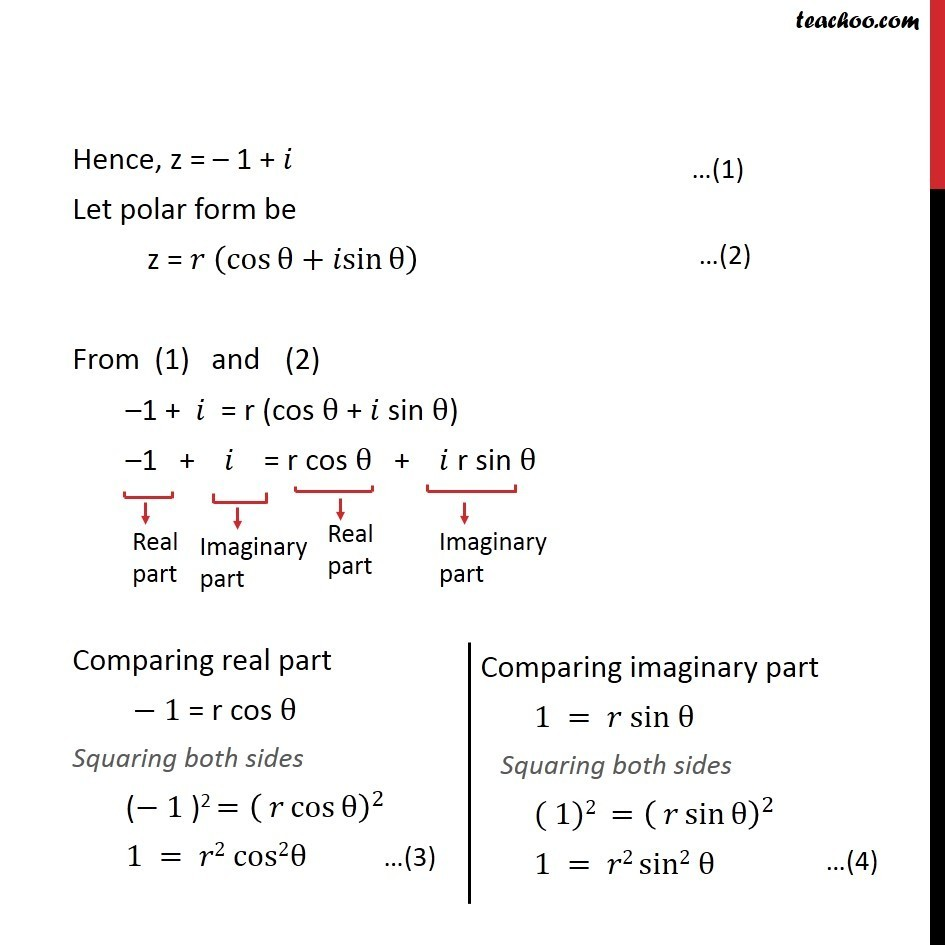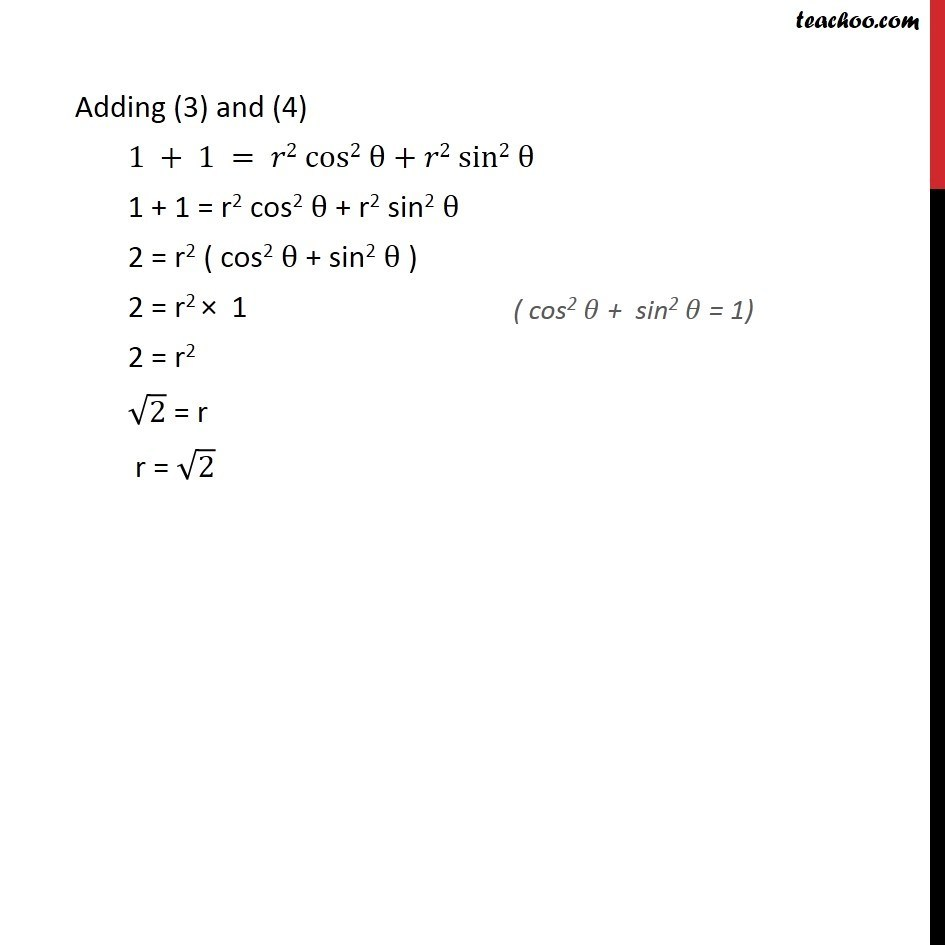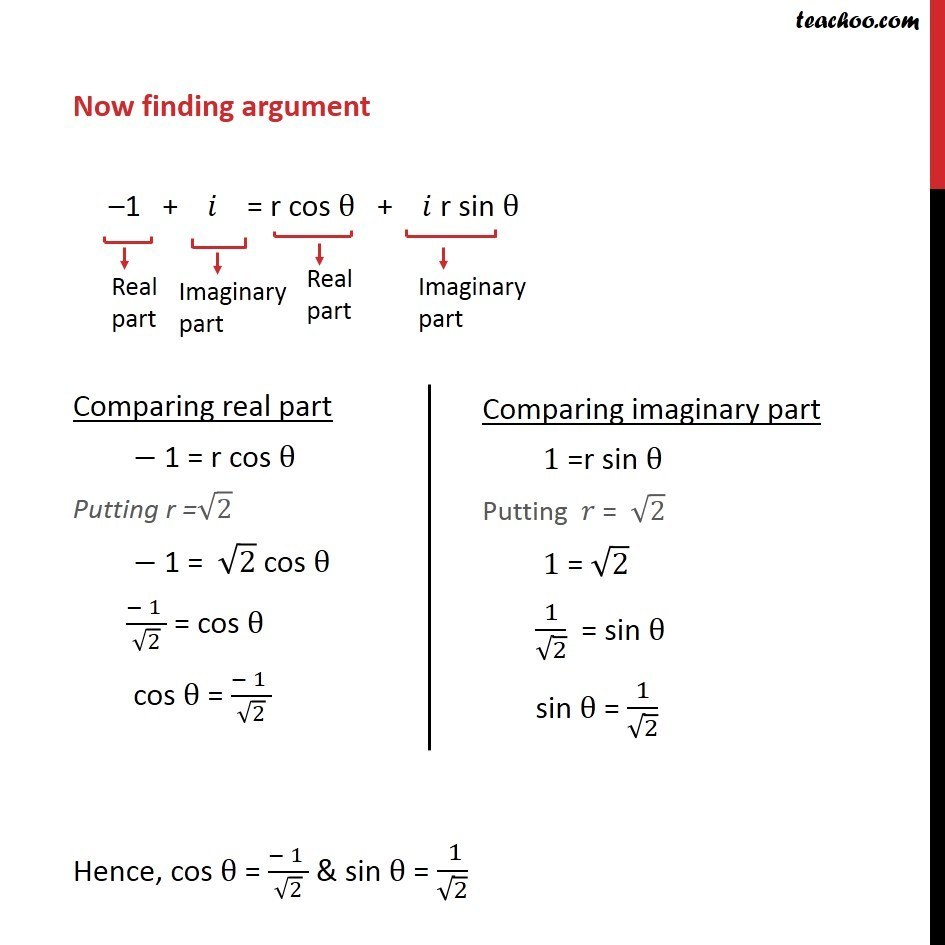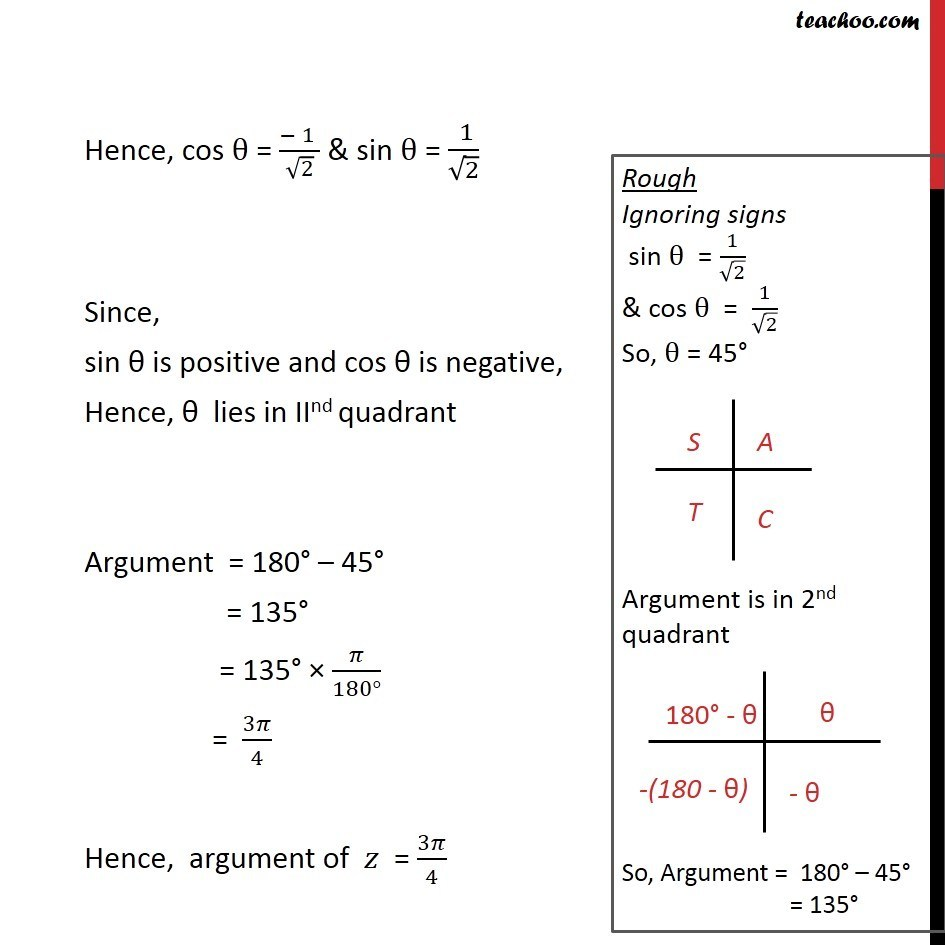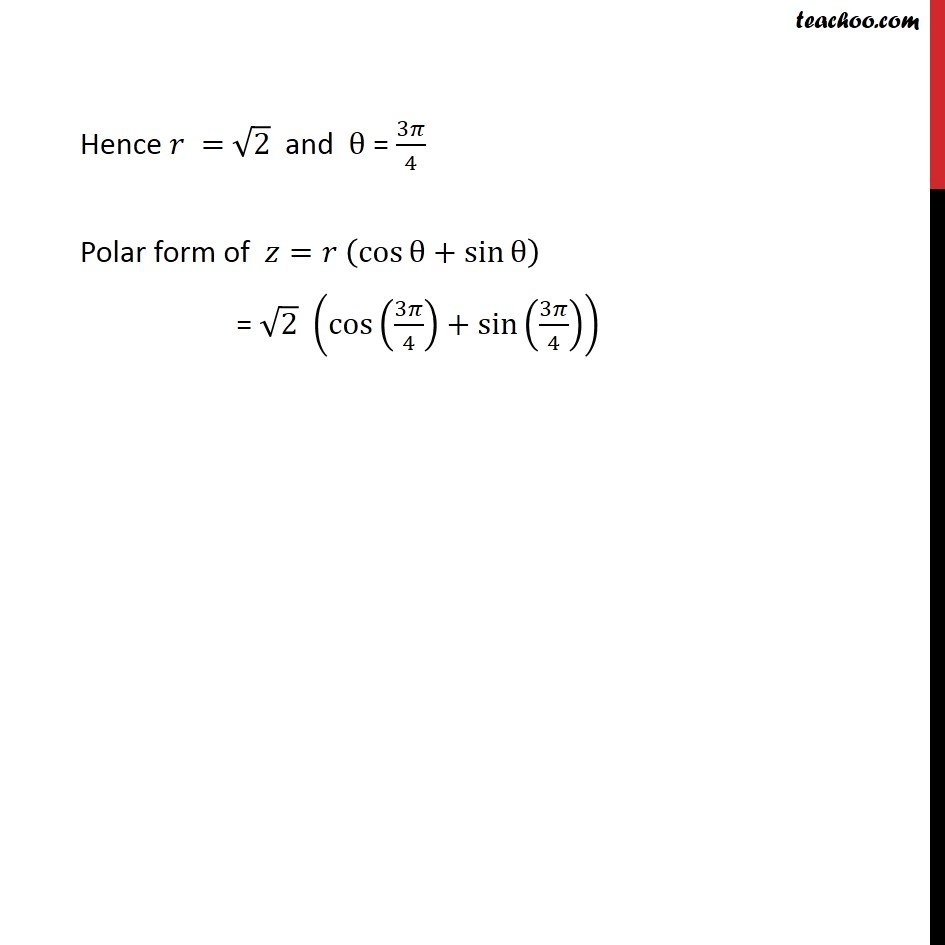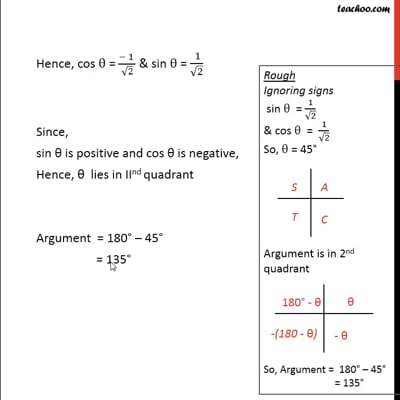This video is only available for Teachoo black users

Introducing your new favourite teacher - Teachoo Black, at only ₹83 per month

### Transcript

Misc 5 Convert the following in the polar form: (ii) ( 1 + 3𝑖)/(1 − 2𝑖) Let z = ( 1 + 3𝑖)/(1 − 2𝑖) Rationalizing = (1 + 3𝑖)/(1 − 2𝑖) × (1 + 2𝑖)/(1 + 2𝑖) = ((1 + 3𝑖) (1 + 2𝑖 ))/((1 − 2𝑖) (1 + 2𝑖)) = (1 (1 + 2𝑖) + 3𝑖 (1 + 2𝑖 ))/((1 − 2𝑖) (1 + 2𝑖)) = (1 +2𝑖 +3𝑖 +6𝑖2)/((1 − 2𝑖) (1 + 2𝑖)) = (1 + 5𝑖 +6𝑖2)/((1 −2𝑖) (1 +2𝑖)) Using (a – b) (a + b) = a2 – b2 = (1 + 5𝑖 +6𝑖2)/((1)2− (2𝑖)2) = (1 + 5𝑖 +6𝑖2)/(1 −4𝑖2) Putting 𝑖2 = –1 = (1 + 5𝑖 +6 (−1 ))/(1 −4 ( −1 )) = (1 + 5𝑖 −6)/(1 +4) = (1 −6 + 5𝑖)/5 = (− 5 + 5𝑖)/5 = (− 5 ( −1 + 𝑖 ))/5 = - 1 + 𝑖 Hence, z = – 1 + 𝑖 Let polar form be z = 𝑟 (cos⁡θ+𝑖sin⁡θ ) From (1) and (2) –1 + 𝑖 = r (cos θ + 𝑖 sin θ) –1 + 𝑖 = r cos θ + 𝑖 r sin θ Comparing real part − 1 = r cos θ Squaring both sides (− 1 )2 =( 𝑟 cos⁡θ )^2 1 = 𝑟2 cos2θ Adding (3) and (4) 1 + 1 = 𝑟2 cos2 θ + 𝑟2 sin2 θ 1 + 1 = r2 cos2 θ + r2 sin2 θ 2 = r2 ( cos2 θ + sin2 θ ) 2 = r2 × 1 2 = r2 √2 = r r = √2 Now finding argument –1 + 𝑖 = r cos θ + 𝑖 r sin θ Comparing real part − 1 = r cos θ Putting r =√2 − 1 = √2 cos θ (− 1 )/√2 = cos θ cos θ = (− 1 )/√2 Hence, cos θ = (− 1 )/√2 & sin θ = ( 1)/√2 Hence, cos θ = (− 1 )/√2 & sin θ = ( 1)/√2 Since, sin θ is positive and cos θ is negative, Hence, θ lies in IInd quadrant Argument = 180° – 45° = 135° = 135° × 𝜋/(180°) = 3𝜋/4 Hence, argument of 𝑧 = 3𝜋/4 Hence 𝑟 = √2 and θ = 3𝜋/4 Polar form of 𝑧=𝑟 (cos⁡θ+sin⁡θ ) = √2 (cos(3𝜋/4)+sin(3𝜋/4))